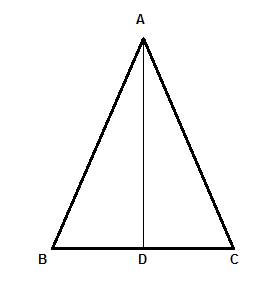Courses
Courses for Kids
Free study material
Free LIVE classes
MoreLIVE
Join Vedantu’s FREE Mastercalss

# In an equilateral $\Delta ABC,AD\bot BC$. Prove that $A{{B}^{2}}+D{{C}^{2}}=\dfrac{5}{4}A{{C}^{2}}.$Verified
362.7k+ views
Hint: Use the property that the altitude divides the triangle into two equal halves. Then use the Pythagoras theorem to both the triangles and hence find the relation between equations to get the desired results.

In the given question we are given an equilateral $\Delta ABC$ where a point D is specified on BC such that $AD\bot BC.$

So this means in the figure below, $\angle ADC=\angle ADB={{90}^{\circ }}$At first we will write the properties of equilateral triangle which are as follows:
i) All the sides are equal.
ii) All the three internal angles are ${{60}^{\circ }}$ .
iii) The altitude, median, angle bisector and perpendicular bisector for each side are all in the same line.
Thus by the property (iii) of an equilateral triangle we can say that the point ‘D’ divides the sides BC into two equal halves.
So we can say that BD = DC.
As AD divides the equilateral $\Delta ABC$ in two congruent right angled triangles we can apply Pythagora's theorem to both parts of equilateral triangle.
So now by using Pythagoras theorem in $\Delta ADB$ we can say,

$A{{D}^{2}}+B{{D}^{2}}=A{{B}^{2}}..........\left( i \right)$

Similarly, by using Pythagoras theorem in $\Delta ADC$, we can say,

$A{{D}^{2}}+D{{C}^{2}}=A{{C}^{2}}..........\left( ii \right)$

BY moving $A{{D}^{2}}$ from left hand to right hand side in equation (ii) we can write it as,

$D{{C}^{2}}=A{{C}^{2}}-A{{D}^{2}}..........\left( iii \right)$

The equation (i) can be written as,

$A{{B}^{2}}=A{{D}^{2}}+B{{D}^{2}}..........\left( iv \right)$

So now adding (iii) and (iv) we get,

$A{{B}^{2}}+D{{C}^{2}}=A{{C}^{2}}+B{{D}^{2}}..........\left( v \right)$

Now by the property of equilateral triangle, that is all the sides are equal, we can say that,

AC = BC ………………(vi)

As we know that point ‘D’ divides ‘BC’ into two equal parts so we can write,

BC = 2BD ……………(vii)

Now in equation (vi) we can substitute the result of (vii) so now we can write it as,

AC = 2BD
Which can be further written as,

$BD=\dfrac{AC}{2}$

Now squaring on both the sides we get,

$B{{D}^{2}}=\dfrac{A{{C}^{2}}}{4}$

Now putting $B{{D}^{2}}=\dfrac{A{{C}^{2}}}{4}$ in equation (v) we get,

\begin{align} & A{{B}^{2}}+D{{C}^{2}}=A{{C}^{2}}+B{{D}^{2}} \\ & \Rightarrow A{{B}^{2}}+D{{C}^{2}}=A{{C}^{2}}+\dfrac{A{{C}^{2}}}{4} \\ \end{align}

Hence we can write,

$A{{B}^{2}}+D{{C}^{2}}=\dfrac{5}{4}A{{C}^{2}}$

Hence proved

Note: Students generally stuck after reading to the equation $A{{B}^{2}}+D{{C}^{2}}=A{{C}^{2}}+B{{D}^{2}}.$

They generally forget to use the property that the altitude, median and angle bisector are aligned in the same line. So they should be careful about it.
Last updated date: 28th Sep 2023
Total views: 362.7k
Views today: 8.62k